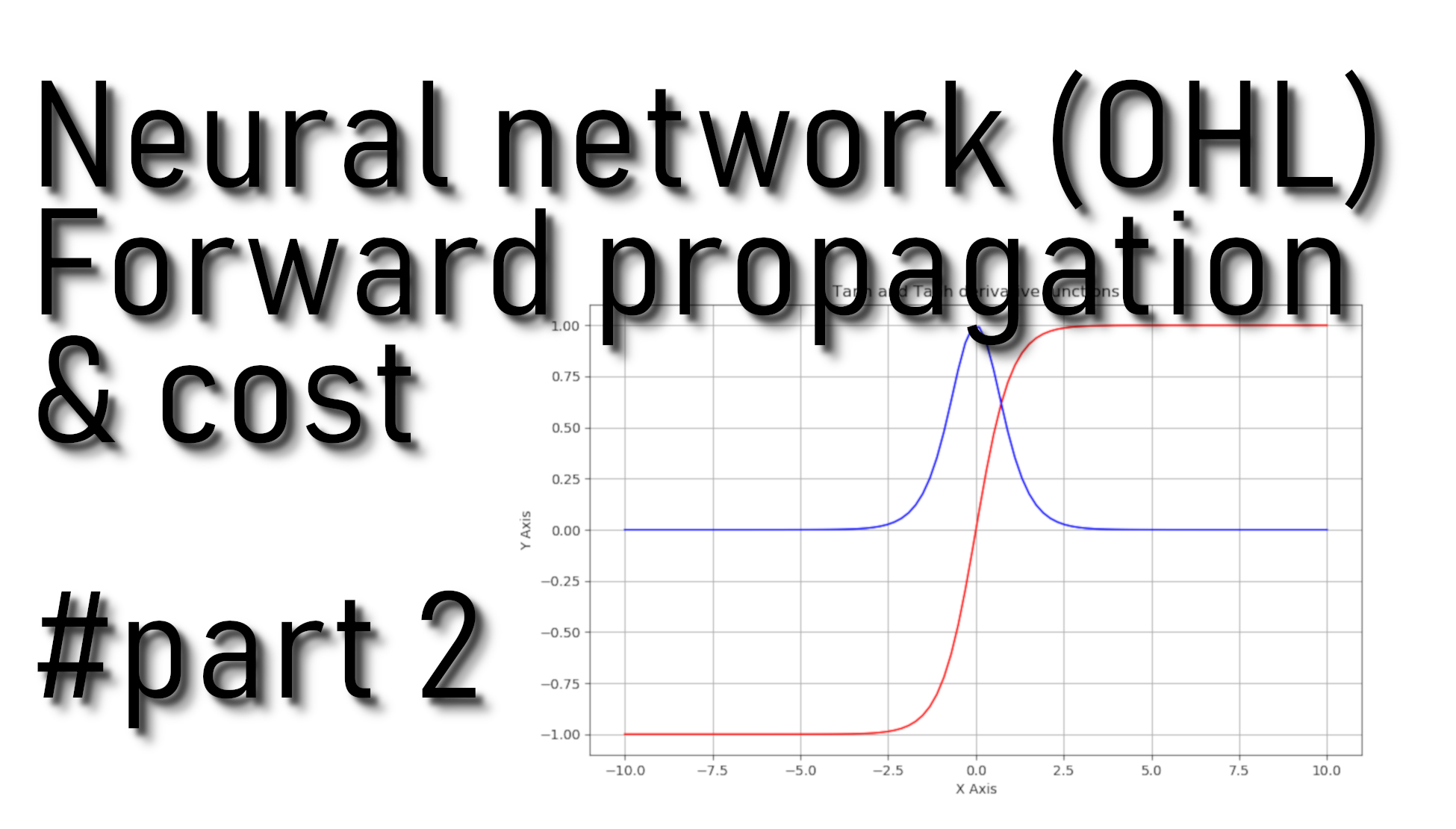# Neural Network forward propagation and cost

## In the previous tutorial, we initialized our model parameters. In this part we'll for this we'll use cache computed during the forward propagation

In the previous tutorial, we initialized our model parameters. In this part, we'll compute forward propagation and cost function. Here are the mathematical expression formulas of forward propagation algorithm for one example x(i) from our previous tutorial part:

${z}^{\left[1\right]\left(i\right)}={W}^{\left[1\right]}{x}^{\left(i\right)}+{b}^{\left[1\right]}\phantom{\rule{0ex}{0ex}}{a}^{\left[1\right]\left(i\right)}=\mathrm{tan}h\left({z}^{\left[1\right]\left(i\right)}\right)\phantom{\rule{0ex}{0ex}}{z}^{\left[2\right]\left(i\right)}={W}^{\left[2\right]}{a}^{\left[1\right]\left(i\right)}+{b}^{\left[2\right]}\phantom{\rule{0ex}{0ex}}\stackrel{^}{y}={a}^{\left[2\right]\left(i\right)}=\sigma \left({z}^{\left[2\right]\left(i\right)}\right)$

So at first, we'll retrieve each parameter from the dictionary "parameters," and then we'll compute Z, A, Z, and A (the vector of all your predictions on all the examples in the training set). Then we'll store values into the cache, which will be used as an input to the backpropagation function.

## Code for our forward propagation function:

Arguments:

X - input data of size (input_layer, number of examples)
parameters - python dictionary containing your parameters (output of initialization function)

Return:

A2 - The sigmoid output of the second activation
cache - a dictionary containing "Z1", "A1", "Z2" and "A2"

def forward_propagation(X, parameters):
# Retrieve each parameter from the dictionary "parameters"
W1 = parameters["W1"]
b1 = parameters["b1"]
W2 = parameters["W2"]
b2 = parameters["b2"]

# Implementing Forward Propagation to calculate A2 probabilities
Z1 = np.dot(W1, X) + b1
A1 = np.tanh(Z1)
Z2 = np.dot(W2, A1) + b2
A2 = sigmoid(Z2)

# Values needed in the backpropagation are stored in "cache"
cache = {"Z1": Z1,
"A1": A1,
"Z2": Z2,
"A2": A2}

return A2, cache

## Computing Neural network cost:

Now that we have computed A (in the Python variable A2), which contains a(i) for every example, we can compute the cost function, which looks like this:

$J=-\frac{1}{m}\sum _{i=1}^{m}\left({y}^{\left(i\right)}\mathrm{log}\left({a}^{\left[2\right]\left(i\right)}\right)-\left(1-{y}^{\left(i\right)}\right)\mathrm{log}\left(1-{a}^{\left[2\right]\left(i\right)}\right)\right)$

## Code for our cost function:

Arguments:

A2 - The sigmoid output of the second activation, of shape (1, number of examples);
Y - "true" labels vector of shape (1, number of examples);
parameters - python dictionary containing parameters W1, b1, W2, and b2.

Return:

cost - cross-entropy cost.

def compute_cost(A2, Y, parameters):
# number of example
m = Y.shape

# Compute the cross-entropy cost
logprobs = np.multiply(np.log(A2),Y) +  np.multiply(np.log(1-A2), (1-Y))
cost = -1/m*np.sum(logprobs)

# makes sure cost is the dimension we expect, E.g., turns [] into 51
cost = np.squeeze(cost)

return cost

## Full tutorial code:

import os
import cv2
import numpy as np
import matplotlib.pyplot as plt
import scipy

ROWS = 64
COLS = 64
CHANNELS = 3

#TRAIN_DIR = 'Train_data/'
#TEST_DIR = 'Test_data/'

#train_images = [TRAIN_DIR+i for i in os.listdir(TRAIN_DIR)]
#test_images =  [TEST_DIR+i for i in os.listdir(TEST_DIR)]

return cv2.resize(img, (ROWS, COLS), interpolation=cv2.INTER_CUBIC)

def prepare_data(images):
m = len(images)
X = np.zeros((m, ROWS, COLS, CHANNELS), dtype=np.uint8)
y = np.zeros((1, m))
for i, image_file in enumerate(images):
if 'dog' in image_file.lower():
y[0, i] = 1
elif 'cat' in image_file.lower():
y[0, i] = 0
return X, y

def sigmoid(z):
s = 1/(1+np.exp(-z))
return s
'''
train_set_x, train_set_y = prepare_data(train_images)
test_set_x, test_set_y = prepare_data(test_images)

train_set_x_flatten = train_set_x.reshape(train_set_x.shape, ROWS*COLS*CHANNELS).T
test_set_x_flatten = test_set_x.reshape(test_set_x.shape, -1).T

train_set_x = train_set_x_flatten/255
test_set_x = test_set_x_flatten/255
'''
#train_set_x_flatten shape: (12288, 6002)
#train_set_y shape: (1, 6002)

def initialize_parameters(input_layer, hidden_layer, output_layer):
# initialize 1st layer output and input with random values
W1 = np.random.randn(hidden_layer, input_layer) * 0.01
# initialize 1st layer output bias
b1 = np.zeros((hidden_layer, 1))
# initialize 2nd layer output and input with random values
W2 = np.random.randn(output_layer, hidden_layer) * 0.01
# initialize 2nd layer output bias
b2 = np.zeros((output_layer,1))

parameters = {"W1": W1,
"b1": b1,
"W2": W2,
"b2": b2}

return parameters

def forward_propagation(X, parameters):
# Retrieve each parameter from the dictionary "parameters"
W1 = parameters["W1"]
b1 = parameters["b1"]
W2 = parameters["W2"]
b2 = parameters["b2"]

# Implementing Forward Propagation to calculate A2 probabilities
Z1 = np.dot(W1, X) + b1
A1 =  np.tanh(Z1)
Z2 = np.dot(W2, A1) + b2
A2 = sigmoid(Z2)

# Values needed in the backpropagation are stored in "cache"
cache = {"Z1": Z1,
"A1": A1,
"Z2": Z2,
"A2": A2}

return A2, cache

def compute_cost(A2, Y, parameters):
# number of example
m = Y.shape

# Compute the cross-entropy cost
logprobs = np.multiply(np.log(A2),Y) +  np.multiply(np.log(1-A2), (1-Y))
cost = -1/m*np.sum(logprobs)

# makes sure cost is in dimension we expect, E.g., turns [] into 51
cost = np.squeeze(cost)

return cost

## Conclusion:

Up to this point, we have initialized our model's parameters, implement forward propagation and compute the loss—few more functions left to write, which we'll continue to do in the next tutorial.